Courses

# Calculus - 2

## 15 Questions MCQ Test Question Bank for GATE Computer Science Engineering | Calculus - 2

Description
This mock test of Calculus - 2 for GATE helps you for every GATE entrance exam. This contains 15 Multiple Choice Questions for GATE Calculus - 2 (mcq) to study with solutions a complete question bank. The solved questions answers in this Calculus - 2 quiz give you a good mix of easy questions and tough questions. GATE students definitely take this Calculus - 2 exercise for a better result in the exam. You can find other Calculus - 2 extra questions, long questions & short questions for GATE on EduRev as well by searching above.
QUESTION: 1

### What is the maximum value of the function f(x) = 2x2 - 2x + 6 in the interval [0, 2]?

Solution:

We need absolute maximum of
f(x) = 2x2 - 2x + 6
in the interval [0, 2]
First find local maximum if any by putting f'{x) = 0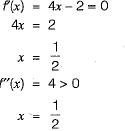is a point of local minimum. So there is no point of local maximum.
Now tabulate the values of f at end point of interval and at local maximum if any (in this case no point of local maximum).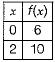Clearly the absolute maxima is at x = 2 and absolute maximum value is 10.

QUESTION: 2

### The following definite integral evaluates to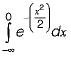Solution: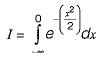Comparing with area under the standared normal curve from -∝ to 0.
We get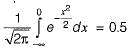So, the required integral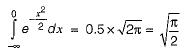QUESTION: 3

### If f(x) is defined as follows, what is the minimum value of f{x) for x ∈ (0, 2]?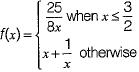Solution:

For the function 25/8x the minimum value will come when x is maximum since it is a decreasing function.
The maximum value of x is 3/2.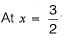the function has the value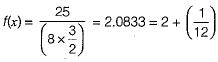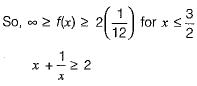But since for this function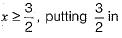this function we get the minimum of this function which is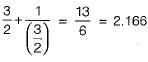Now comparing the minimum value 2.0833 of the first function with minimum value 2.166 of the second function, we get the overall minimum of this function to be 2.0833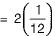which is option (b).

QUESTION: 4

A point on a curve is said to be an extremum if it is a local minimum or a local maximum. The number of distinct extrema for the curve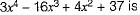Solution: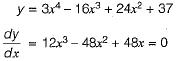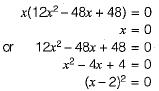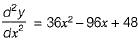Now
x = 0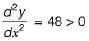∴ f(x) has a minimum at x = 0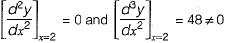So x = 2 is a saddle point (point of inflection)
∴ f(x) has no extremum at x = 2. So f(x) has only one point of extremum (at x = 0).

QUESTION: 5

The value of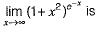Solution: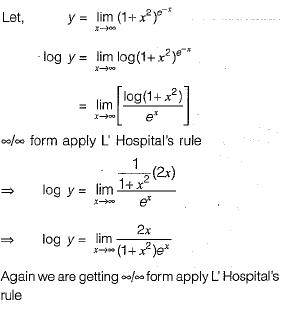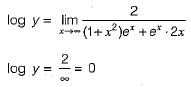⇒ y = 1

QUESTION: 6

If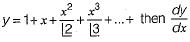is equal to

Solution: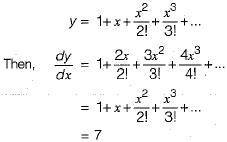QUESTION: 7

If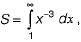then S has the value

Solution: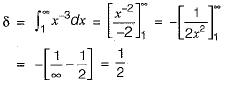QUESTION: 8

Consider function f(x) = (x2- 4)2 where x is a real number. Then the function has

Solution: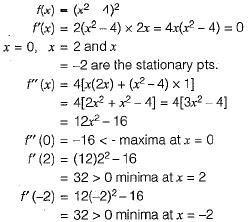∴ There is only one maxima and only two minima for this function.

QUESTION: 9

The value of the quantity P, where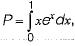is equal to

Solution: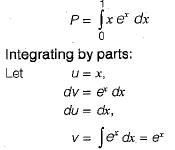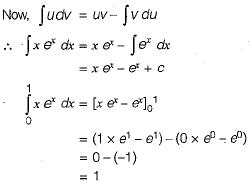QUESTION: 10

The function f(x) = 2x - x2 + 3 has

Solution: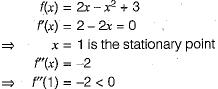So at x = 1 we have a relative maxima.

QUESTION: 11

The maximum value of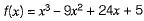in the interval [1, 6] is

Solution:

We need absolute maximum of
f(x) = x3 - 9x2 + 24x + 5 in the interval [1,6]
First find local maximum if any by putting f'(x) = 0.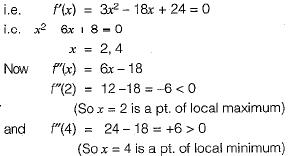Now tabulate the values of f at end pt. of interval and at local maximum pt., to find absolute maximum in given range, as shown below: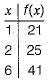Clearly the absolute m axim a is at x = 6 and absolute maximum value is 41.

QUESTION: 12

Let f(x) = x e-x. The maximum value of the funntion in the interval (0, ∝) is

Solution: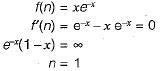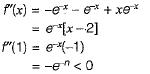Hence f(n) have maximum value at n = 1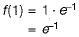Let,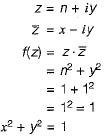QUESTION: 13

Minimum of the real valued function f(x) = (x-1)2/3 occurs at  x equal to

Solution: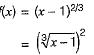As f(x) is square of hence its minimum value be 0 where at x = 1.

QUESTION: 14

The minimum value of the function f(x) = x3-3x2 - 24x + 100 in the interval [-3, 3] is

Solution: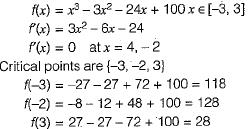Hence f(x) has minimum value at x = 3 which is 28.

QUESTION: 15

If a continuous function f(x) does not have a root in the interval [a, b], then which one of the following statements is TRUE?

Solution:

Intermediate value theorem states that if a function is continuous and f(a) • f(b) < 0, then surely there is a root in (a, b). The contrapositive of this theorem is that if a function is continuous and has no root in (a, b) then surely f(a) . f{b) ≥ 0. But since it is given that there is no root in the closed interval [a ,b] it means f(a) . f(b) ≠ 0.
So surely f(a) . f(b) > 0.